# Miniatures figurinesModel: Mf01

Dimensions:

Length x breadth x height: 10 x 4 x 6 cm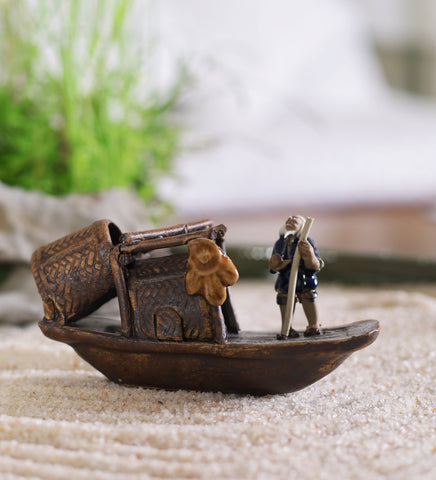Model: Mf02

Dimensions:

Length x breadth x height: 10 x 4 x 6 cm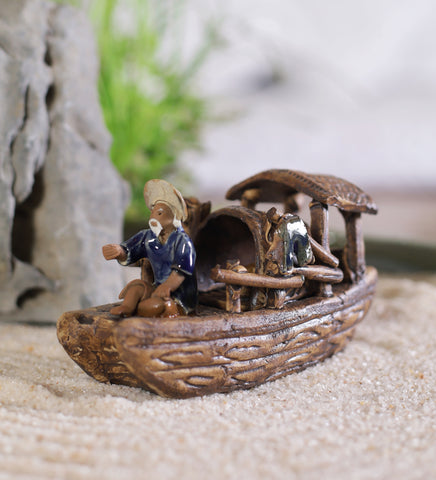Model: Mf03

Dimensions:

Length x breadth x height: 10 x 4 x 4.5 cm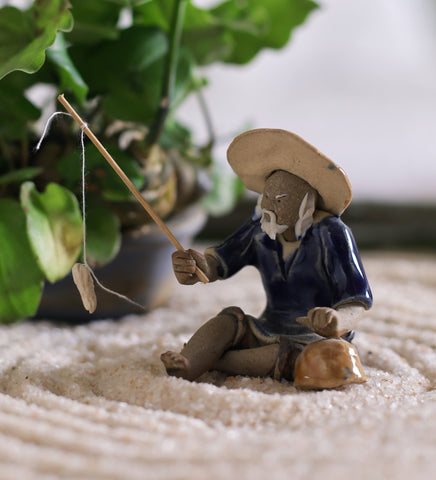Model: MF04

Dimensions:

A: 5 x 6 x 7 cm

B: 4 x 5 x 6 cmModel: MF05

Dimensions:

Length x breadth x height: 9 x 9 x 12 cmModel: MF06

Dimensions:

Length x breadth x height: 8 x 6 x 8 cmModel: MF07

Dimensions:

Length x breadth x height: 8 x 6 x 8 cm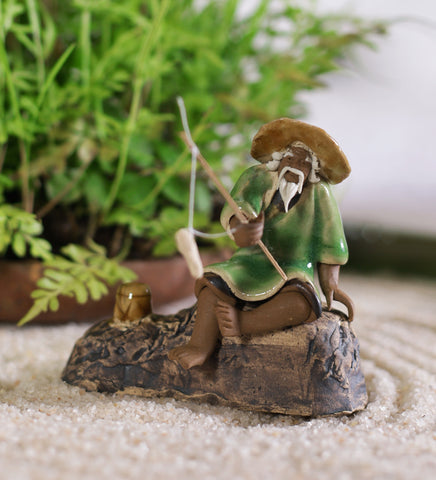Model: MF08

Dimensions:

Length x breadth x height: 8 x 6 x 8 cm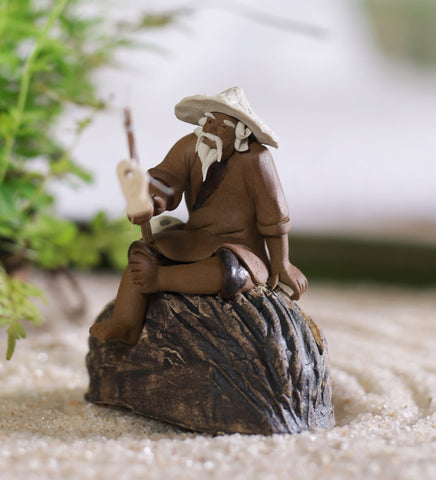Model: MF09

Dimensions:

Length x breadth x height: 8 x 6 x 8 cm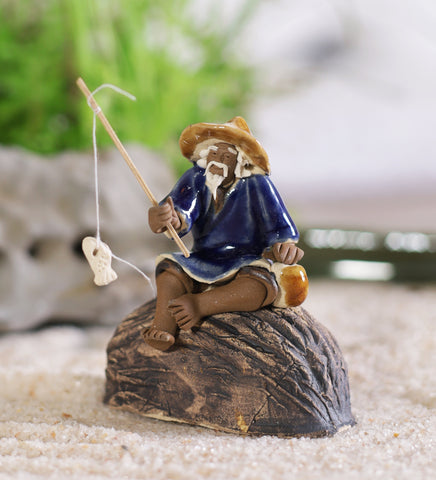Model: MF10

Dimensions:

Length x breadth x height: 8 x 6 x 8 cmModel: MF11

Dimensions:

21 x 12 x 11 cm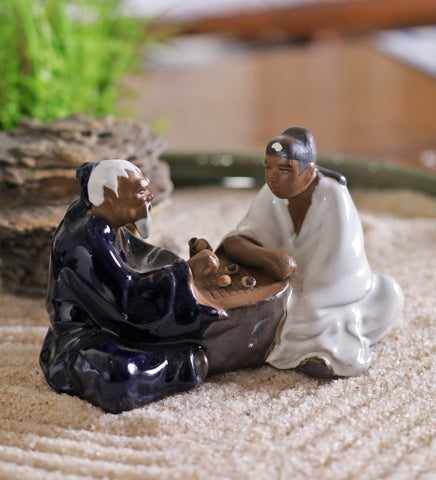Model: MF12

Dimensions:

Length x breadth x height:  15 x 8 x 9 cmModel: MF13

Dimensions:

Length x breadth x height: 8 x 6 x 11 cm